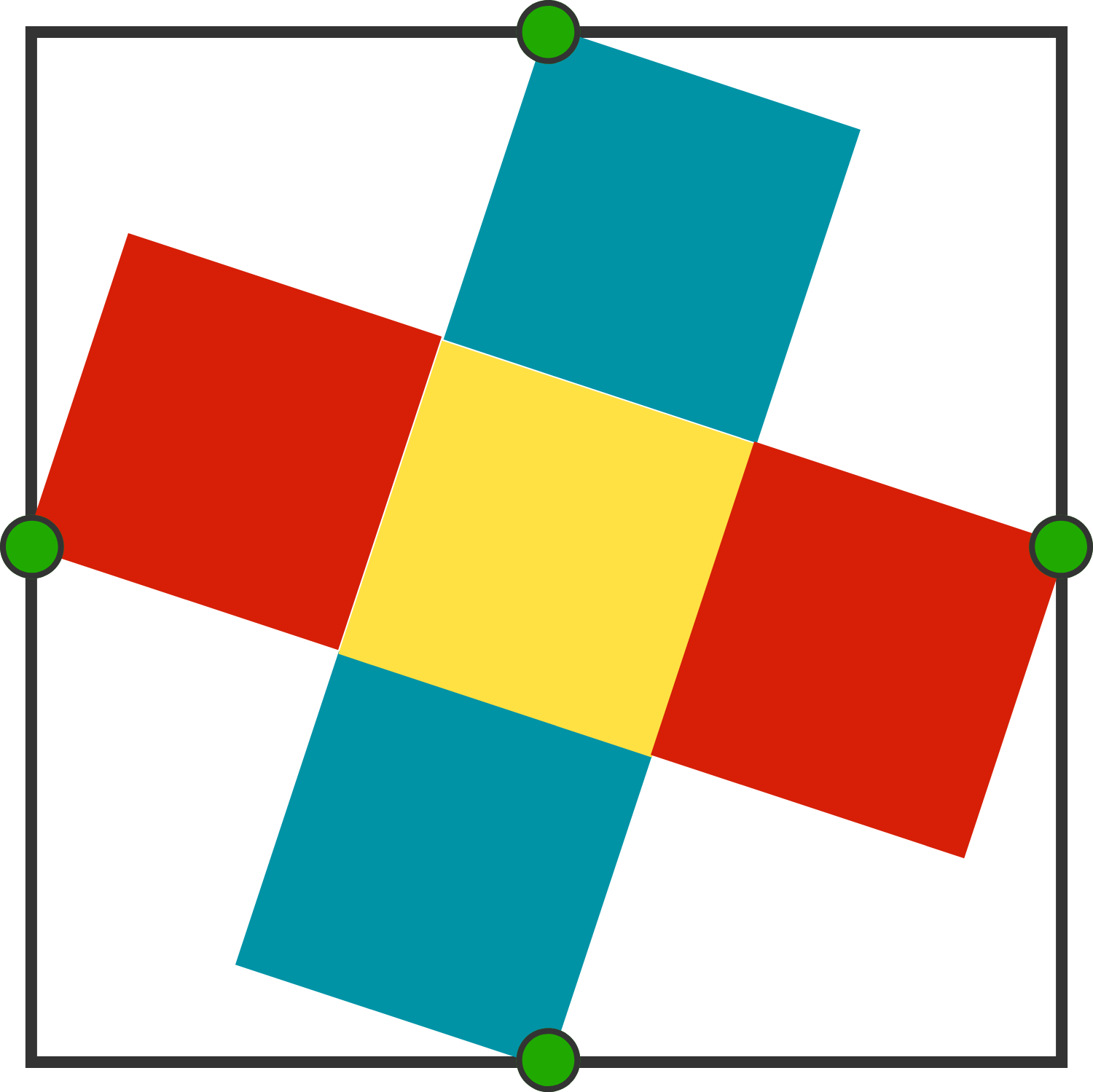# Cross

Geometry Level 1

We inscribed five congruent, small squares into a larger square, as shown in the figure below. The green points are the midpoints of the large square's sides.

If a small square has area $10,$ what is the area of the large square?×